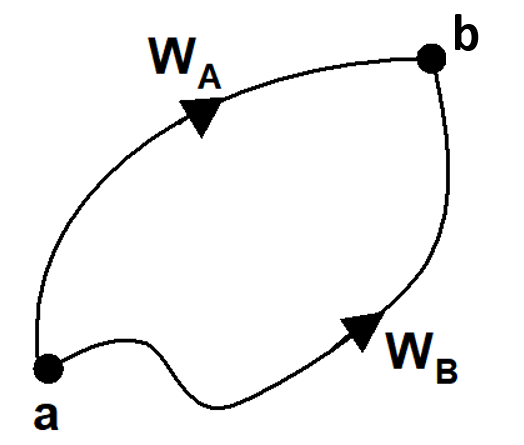Most Affordable JEE | NEET | 8,9,10 Preparation by Kota's Top IITian Doctor Faculties

# Conservative and Non Conservative forces - Physics - eSaral`

Hey, do you want to learn about the Conservative and Non-Conservative forces? if so. Then you are at the right place.

## Conservative force

A force is said to be conservative if the work done by or against the conservative force :
(a) Is independent of path and depends only on initial and final positions
(b) Does not depend on the nature of path followed between the initial and final positions.

### Examples of Conservative Force

All central forces are conservative like gravitational, electrostatic, elastic force, restoring force due to spring etc.

In presence of conservative forces, mechanical energy remains constant.

### To summarize with

1. When force or more than one forces are acting on a particle in such a way that if particle come back to its initial position and kinetic energy of particle is equal to its previous value which was initially, than forces are known as conservative forces.
2. A force is conservative if the work done by it on a particle that moves between two points a and b along two differents paths A and B. Such that $W_{A}=W_{B}$.
3. If work done by a force acting on a particle over a close path is zero then force is conservative $W_{a b}+W_{b a}=0$ or $W_{a b}=-W_{b a}$.

These three defination are statements of the same thing in different way.Special Point :

1. Work done along a closed path or in a cyclic process is zero. i.e. $\oint F .dx = 0$

(b) If $\overrightarrow {\rm{F}}$ conservative force then $\vec \nabla \times \vec F = 0$.

## Central Forces

The force whose line of action always passes through a fixed point (which is known as centre of force) and magnitude of force depends only on the distance from this point is known as central force.

$\overrightarrow {\rm{F}}$= F(r) (±) $\widehat {\bf{r}}$ = ± F(r) ${{\vec r} \over r}$

All forces following inverse square law are central forces.

$\overrightarrow {\rm{F}} = {{\rm{k}} \over {{{\rm{r}}^2}}}\widehat {\rm{r}}$ is central force like Gravitational force and Columb force.

If central force act on a particle then torque acting on the particle is

$\vec \tau = \vec r \times \vec F = \vec r \times F(r)\hat r$

$= \vec r \times F(r){{\vec r} \over r} = (\vec r \times \vec r){{F(r)} \over r}$ (because $\vec r \times \vec r = 0$

because $\vec \tau = 0$

$\vec \tau = {{\overrightarrow {\rm{d}} {\rm{J}}} \over {{\rm{dt}}}}$ because ${{{\rm{d}}\overrightarrow {\rm{J}} } \over {{\rm{dt}}}} = 0$ $\overrightarrow {\rm{J}}$ = constant

When a particle moves under the action of central force then its angular momentum remains conserved.

1. All central forces are conservative forces

2. Central forces are function of position

3. The angular momentum of an object moving under a central force is conserved

## Non-conservative force

A force is said to be non-conservative if work done by or against the force in moving a body depends upon the path between the initial and final positions.

Work done in a closed path is not zero in a non-conservative force field.

The frictional forces are non-conservative forces. This is because the work done against friction depends on the length of the path along which a body is moved. It does not depend only on the initial and final positions. Note that the work done by frictional force in a round trip is not zero.

### Examples of non-conservative force

The velocity-dependent forces such as air resistance, viscous force etc. are non-conservative forces.

## Difference Between Conservative and Non-Conservative forces

For a better understanding of this chapter, please check the detailed notes of Work, Energy, and Power. If you want more Free Learning Videos and Study Material Then don't forget to download the eSaral App.